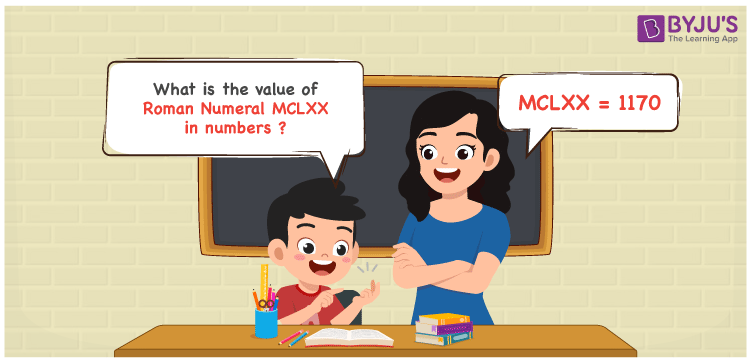Checkout JEE MAINS 2022 Question Paper Analysis : Checkout JEE MAINS 2022 Question Paper Analysis :

# MCLXX Roman Numerals

MCLXX Roman Numerals is 1170. This article gives a complete knowledge of how MCLXX is written in numbers. Students will be able to convert the roman numerals to numbers and vice versa if these articles are understood well. These roman numerals conversion materials at BYJU’S are absolutely free of cost so that students can get it handy while learning new topics. Therefore, MCLXX can be indicated as 1170 in numbers.

 Number Roman Numeral 1170 MCLXX## How to Write MCLXX Roman Numerals in Numbers?

Students will learn the process to convert MCLXX to numbers referring to the steps here.

MCLXX = M + C + L + X + X

MCLXX = 1000 + 100 + 50 + 10 + 10

MCLXX = 1170

## Video Lesson on Roman Numerals## Frequently Asked Questions on MCLXX Roman Numerals

### Why should 1170 be written as MCLXX?

In roman numerals

1000 is written as M

100 is written as C

70 is written as LXX

1170 = M + C + LXX = MCLXX

### Calculate 1000 + 170.

We know that

1000 + 170 = 1170

Hence, 1000 + 170 is 1170 which is written as MCLXX.

### Determine the remainder we get when MCLXX is divided by IV.

We know that

MCLXX = 1170

IV = 4

So the remainder we get when MCLXX is divided by IV is II.